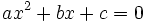# Linear, Non-Linear, Differential, and Quadratic Equations: Oh My!

Article Summary: Do you see any comparisons between the different types of equations? Not really, they are all used to calculate different things. Linear equations are used for straight lines on a graph. None-linear are used for determining the how non-straight things happen the way they do. Differential equations are to explain changes in nature. Finally, quadratic equations are used to explain curves.

We are going to look at linear, non-linear, differential, and quadratic equations to why they are different and to see if there are any similarities. First let's take a look at linear equations.

A linear equation is a form of algebraic equation that is used to calculate the slope of a straight line. The results of the calculations are then plotted on a Cartesian grid using the coordinates derived from the calculation. The most common formula used in for linear equations is y = mx + b. The point that is plotted on the Cartesian grid is determined by the constant "m" that determines the slope and the constant "b" will determine where the straight line crosses the "y" axis on the grid.

Linear equations can also be used to make predictions on the outcome of certain events. For example: you want to have a yard sale to get rid of some of your old toys, video games, and other things. You will look at your items and try to determine how much you will make. You plot points on the graph based on your sales and then you can predict how much you will make if sells continue the same.

Linear equations are used to find the slope on a graph. This is handy when you add specific properties to the "x" and "y" axis. Now you can pick any point on the line and find the outcome at the intersection of the "x" and "y" axis. This is handy for making predictions and is used in all forms of sports and business.

A non-linear equation is not a straight line and is essentially difficult to solve. They are used primarily by mathematicians and scientists. They use this type of equation to explain natural occurring events, because very few things in life occur in a strait line. I you have ever heard of the chaos theory, non-linear equations are used to attempt to explain chaos.

Differential equations are used primarily in science and technology, where relationships between events are continuously changing. For example, Newton's Laws of Motion that explains the position, velocity, acceleration, and forces that act on objects to make them move, stay in motion, or rest. There are different types of differential equations:

• Ordinary - uses one single independent variable
• Partial - uses multiple independent variables

Differential equations are used to calculate how a leopard gets its spots. Describing how diseases spread throughout a region or country. In addition it is used by meteorologists to explain the patterns in weather. Differential equations are also used in the development of video games to predict and control specific actions within the game.

Quadratic equationsare used to find the curve on a Cartesian grid. They are primarily used to find the curve that objects take when the fly through the air. For example a football, baseball, soccer ball, basketball, etc. They also used to design any object that has curves that must have a particular curved shape. The military uses the quadratic equation when they want to predict where artillery shells hit when fired from cannons. The quadratic equation is also used to explain how the planets in our solar system revolve around the sun.

Do you see any comparisons between the different types of equations? Not really, they are all used to calculate different things. Linear equations are used for straight lines on a graph. None-linear are used for determining the how non-straight things happen the way they do. Differential equations are to explain changes in nature. Finally, quadratic equations are used to explain curves.